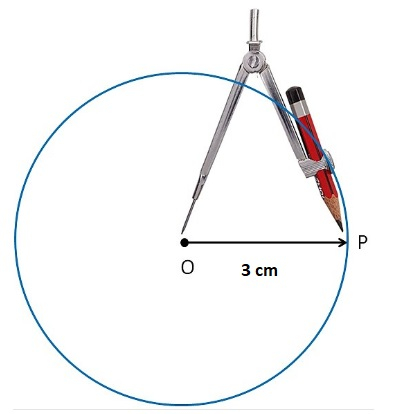# Construct a circle with a radius of 3 cm.

Given :

The radius of the circle is 3 cm.

To do :

We have to construct a circle with a radius of 3 cm.

Solution :

To draw a circle whose radius is given, we use a ruler and compasses. Given that the radius is 3 cm, for example, the steps to be followed are:

1: Place the pointer of the compass at the initial point of the ruler (0 cm) and extend the other end of the pencil measuring 3 cm from the initial point (i.e. 3 cm)

2: Mark a point O on a piece of paper. This point is the center of the circle that you are about to construct.

3: Place the pointer of the compass at point O.

4: Turn the compass slowly through 360 degrees to draw the required circle.Updated on: 10-Oct-2022

92 Views